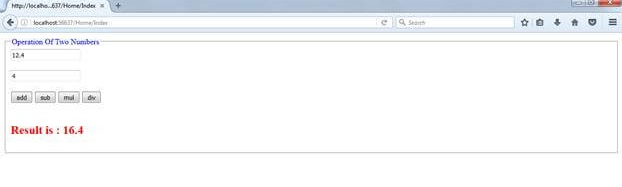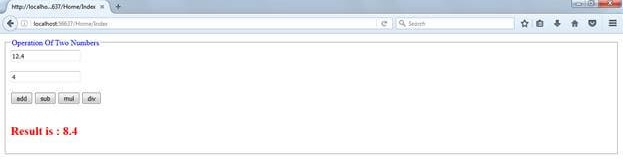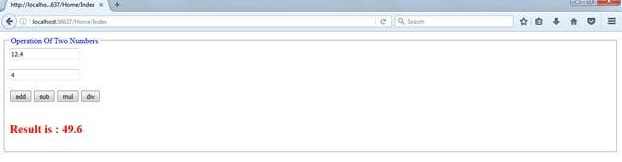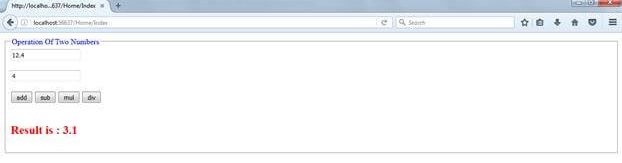{{announcement.body}}
{{announcement.title}}

# Basic Web Calculator in ASP.NET MVC 5

DZone 's Guide to

# Basic Web Calculator in ASP.NET MVC 5

### In this post, we'll learn how to perform an arithmetic operation (Addition, Subtraction, Multiplication, Division) using multiple submit buttons in MVC.

· Web Dev Zone ·
Free Resource

Comment (1)

Save
{{ articles.views | formatCount}} Views

Create Addition, Subtraction, Multiplication, Division Application Using ASP.NET MVC 4.0 With Multiple Submit Buttons.

## Introduction

The MVC architectural pattern separates the user interface (UI) of an application into three main parts.

1. The Model − A set of classes that describes the data you are working with as well as the business logic.
2. The View − Defines how the application’s UI will be displayed. It is pure HTML, which decides how the UI is going to look.
3. The Controller − A set of classes that handles communication from the user, overall application flow, and application-specific logic.

## MVC Description

Thi is responsible for rendering the user interface, whether that be HTML or whether it actually be UI widgets on a desktop application. The view talks to a model, and that model contains all of the data that the view needs to display. Views generally don't have much logic inside of them at all.

The controller that organizes is everything. When an HTTP request arrives for an MVC application, that request gets routed to a controller, and then it's up to the controller to talk to either the database, the file system, or the model.

## Merits Of MVC

• Provides better support for test-driven development (TDD).

• Works well for web applications that are supported by large teams of developers and for web designers who need a high degree of control over the application behavior.

• Direct control over HTML also means better accessibility for implementing compliance with evolving Web standards.

• Makes it easier to manage complexity by dividing an application into the model, the view, and the controller.

## Project Description

In this post, I will show how to perform an arithmetic operation (Addition, Subtraction, Multiplication, Division) using multiple submit buttons in MVC.

### Steps to Be Followed

Step 1: Create an MVC application named "Operation." I named mine "Addition."

Step 2: By default, Visual Studio created some folders for you, like Controllers, Models, Views, etc.

Step 3: Now, go to the "Models" folder and create a class file. Here, we define some entities for accessing the values through it and showing the output.

Step 4: Code Ref. for AdditionViewModel.cs

``````using System;
using System.Collections.Generic;
using System.Linq;
using System.Web;
//public int A { get; set; }
//public int B { get; set; }
//public int Result { get; set; }
public double A {
get;
set;
}
public double B {
get;
set;
}
public double Result {
get;
set;
}
}
}   ``````

### Code Description

Here I used three entities to access input values using A and B given by the user and show the arithmetic operation result using the `Result` variable.

``````public double A {
get;
set;
}
public double B {
get;
set;
}
public double Result {
get;
set;
} ``````

Step5: Then, go to "Controllers" folder and create a Controller class file.

Step6: Code Ref. For HomeController.cs

``````using Addition.Models;
using System;
using System.Collections.Generic;
using System.Linq;
using System.Web;
using System.Web.Mvc;
public class HomeController: Controller {
//
// GET: /Home/
[HttpGet]
public ActionResult Index() {
return View();
}
[HttpPost]
public ActionResult Index(AdditionViewModel model, string command) {
model.Result = model.A + model.B;
}
if (command == "sub") {
model.Result = model.A - model.B;
}
if (command == "mul") {
model.Result = model.A * model.B;
}
if (command == "div") {
model.Result = model.A / model.B;
}
return View(model);
}
}
}   ``````

### Code Description

In the `ActionResult` method, I passed one string parameter named `command`and, using this command, I assigned some arithmetic operation string valuse and based on that the operation will be performed.

``````    public ActionResult Index(AdditionViewModel model, string command) {
model.Result = model.A + model.B;
}
if (command == "sub") {
model.Result = model.A - model.B;
}
if (command == "mul") {
model.Result = model.A * model.B;
}
if (command == "div") {
model.Result = model.A / model.B;
}
return View(model);   ``````

Step7: Go to the "Views" folder and create a folder called "Home" inside of which we'll create a cshtml file named Index.cshtml.

Step8: Code Ref. for Index.cshtml

``````@model Addition.Models.AdditionViewModel
@ {
Layout = null;
}
@using(Html.BeginForm()) { < fieldset > < legend style = "color:blue" > Operation Of Two Numbers < /legend>
@Html.EditorFor(x => x.A) < br / > < br / > @Html.EditorFor(x => x.B) < br / > < br / > < input type = "submit"
name = "command" / > < input type = "submit"
value = "sub"
name = "command" / > < input type = "submit"
value = "mul"
name = "command" / > < input type = "submit"
value = "div"
name = "command" / > < br / > < br / > < h2 style = "color:red" > @Html.Label("Result is :")
@Html.DisplayFor(x => x.Result) < /h2> < /fieldset>
}  ``````

### Code Description

To access input values I need textboxes.

``@Html.EditorFor(x => x.A) < br / > < br / > @Html.EditorFor(x => x.B)  ``

Here I used a reference to the controller class that is command and related string values like add, mul, sub, and div. The button type is submit.

By using multiple submit button type, we can perform arithmetic operations.

``````<input type = "submit"
name = "command" / > < input type = "submit"
value = "sub"
name = "command" / > < input type = "submit"
value = "mul"
name = "command" / > < input type = "submit"
value = "div"
name = "command" / > < br / > < br / > < h2 style = "color:red" > @Html.Label("Result is :")
@Html.DisplayFor(x => x.Result)  ``````

Finally, for showing the resulting output.

``@Html.DisplayFor(x => x.Result)  ``

Step9: Then, go to the "App_Start" folder and open the RouteConfig.cs file to add some code to set the start page when the application will load the first time, like ASP.NET.

Code Ref. for RouteConfig.cs

``````    using System;
using System.Collections.Generic;
using System.Linq;
using System.Web;
using System.Web.Mvc;
using System.Web.Routing;
public class RouteConfig {
public static void RegisterRoutes(RouteCollection routes) {
routes.IgnoreRoute("{resource}.axd/{*pathInfo}");
routes.MapRoute(name: "Default", url: "{controller}/{action}/{id}", defaults: new {
controller = "Home", action = "Index", id = UrlParameter.Optional
});
}
}
}     ``````

### Code Description

Here the controller name is `Home` and the controller action method name is `Index`. So here I'll put a new controller action method, and name it `Index1`.

Then the start page should come with ..../Home/Index1 . So, now, the URL must be ..../Home/Index.new

``````public static void RegisterRoutes(RouteCollection routes) {
routes.IgnoreRoute("{resource}.axd/{*pathInfo}");
routes.MapRoute(name: "Default", url: "{controller}/{action}/{id}", defaults: new {
controller = "Home", action = "Index", id = UrlParameter.Optional
}); ``````

### Output

When the page loads the URL should be like this.

See the URL - http://localhost:56637/Home/Index. Here, "Home" is the Controller name and "Index" is the controller action method name.For SubtractionFor MultiplicationFor Division## Summary

1. The relationship between Controller, Model, View.
2. By using this application your can perform + , - , * , /  on two numerical.
Topics:
mvc ,c# ,visual studio 2015 ,web dev

Comment (1)

Save
{{ articles.views | formatCount}} Views

Opinions expressed by DZone contributors are their own.